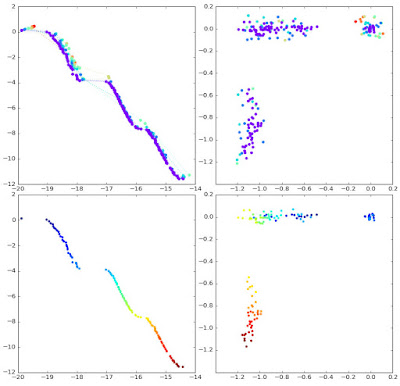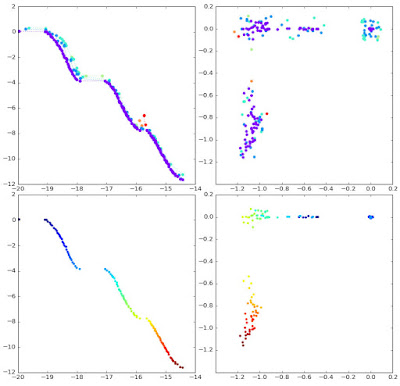Saturday, 14 October 2017

multi-objective optimisation II

In a previous post, I described what a Pareto front is, and Deb et al (2000)’s algorithm for finding it.  We have the first element we need for a multi-objective optimisation algorithm.  There is one more subtlety first, though.

We want to get a good estimate of the whole Pareto front, not just a few points on it.  So we want the points we find to be well spread out across the front.  Deb et al define the crowding distance: we prefer less crowded to more crowded points, as the densely crowded ones are not giving us much extra information about the front.  Their algorithm is:

Here, I is the set of points, and I[i].m is the value of the mth objective function of the ith individual in I.  We can code this up in python as follows (with I being a list of points in a particular front):
def crowding_distance_assignment_single_front(pfront):
# pfront = list of individuals, dictionary { 'state':s, 'fitness':(f1, ... fm) }
inf = 1000000                  # "infinity"
dist =  * len(pfront)       # list of distances, I_distance
m = len(pfront['fitness'])  # no of objectives (= size of 'fitness' tuple)
for mi in range(m):
# sifront = [(i, pfront[i]), ...] sorted on mi-th fitness values of pfront
# sifront[j] = original index i of item in pfront list
# sifront[j]['fitness'][mi] = mi-th fitness value of jth sorted individual
sifront = sorted(enumerate(pfront), key=lambda x:x['fitness'][mi])

dist[sifront] += inf    # first boundary item in list
dist[sifront[-1]] += inf   # last boundary item in list
for i in range(1,len(sifront)-1):
dist[sifront[i]] += sifront[i+1]['fitness'][mi] \
- sifront[i-1]['fitness'][mi]

# sort pfront into reverse distance order, using distance list
dist, pfront = (list(t) for t in
zip(*sorted(zip(dist,pfront), key=lambda x:x, reverse=True)))

return(pfront)
The algorithm works by sorting the list of individuals in order of their fitness value for objective mi.  The distance value of each point is the sum of the distances to its two neighbouring points: crowded points will have low distances, so low distances are bad.  The first and last points in the list are given a very high (good) value.  This is repeated for each objective dimension, adding up the distance measures.

The python then does one more thing than the Deb et al algorithm.  It uses the distances calculated to sort the original list of individuals into reverse distance order (high distance isolated points near the the front, low distance crowded points towards the end) and returns that sorted list.

Then we can sort the list of fronts in the obvious way:
def crowding_distance_assignment(pfrontlist):
for i, pfront in enumerate(pfrontlist):
pfrontlist[i] = crowding_distance_assignment_single_front(pfront)
return(pfrontlist)

Now we have enough to make a multi-objective search algorithm:
Np = 100        # parent population size
Nq = 100        # no of children
Ngen = 250      # no of generations
Nstate = 3      # size of individual state

parents = init_popl(Np,Nstate)
children = make_children(parents, Nq)

for _ in range(Ngen):
popl = parents + children
# construct the list of fronts from the population (previous post)
pfrontlist = fast_nondominated_sort(popl)
# sort each front into least crowded (highest distance) order
pfrontlist = crowding_distance_assignment(pfrontlist)

# new parents become the best of the previous popl of parents + children
parents = [item for pfront in pfrontlist for item in pfront][:Np]
# make new children from those parents
#  note that parents are sorted in fitness order
children = make_children(parents, Nq)

We need to define init_popl() to build some initial (usually random) population.
import random
def init_popl(n,nstate):
# return list of n individuals, each with nstate state vars, each in -1..1
pop = []
for _ in range(n):
state = tuple([random.uniform(-1,1.) for i in range(nstate)])
pop += [{'state': state, 'fitness': fitness(state)}]
return pop
Here I have simply hardcoded in an initial range of +/-1, because I am demonstrating this with the equally hard-coded Kursawe function objectives:
import math
def fitness(state):
# Kursawe function
x1,x2 = state
f1 = -10*math.exp(-0.2*math.sqrt(x1**2+x2**2)) \
- 10*math.exp(-0.2*math.sqrt(x2**2+x3**2))
f2 = abs(x1)**0.8+5.*math.sin(x1**3) \
+ abs(x2)**0.8+5.*math.sin(x2**3) \
+ abs(x3)**0.8+5.*math.sin(x3**3)
return(f1,f2)
Now all that’s left is to define make_children(), which breeds the next generation. Deb et al simply say “Binary tournament selection, recombination, and mutation operators are used to create a child population”.  So, for the sake of demonstration, let’s do the simplest possible thing here: binary tournament with a fixed mutation of the three component state, and no recombination:
def make_children(parents, nq):
# parents = list of items in fitness order, fittest first
#   (except on v first call)
# nq = no of children to make

# tournament selection + mutation
np = len(parents)
children = []
for _ in range(nq):
# binary tournament: chose two parents randomly
p12 = random.sample(range(np),2)
# tournament winner = lower number, because sorted
p = parents[min(p12)]
s = mutate(p['state'])
children += [ {'state': s, 'fitness': fitness(s)} ]
return children

def mutate(state):
x,y,z = state
return (x+random.uniform(-0.1,0.1),
y+random.uniform(-0.1,0.1),
z+random.uniform(-0.1,0.1))

Clearly, init_popl(), fitness(), make_children(), and mutate() should be modified into general purpose code, but that’s not the point of this post.

Here are some results, for the Kursawe function, and for a population size of 100 (click to get a larger view).After 2 generations: (top left) fitness/objective values (f1,f2), with the current fronts of the entire population as calculated using fast_nondominated_sort(), and different front plotted in different colours; (top right) the corresponding (x1,x2) state space (x3 omitted for clarity) of the entire population, with the points plotted in the same colours as in the fitness plot; (bottom left) the fittest front only, plotted with a rainbow colouring along its length; (bottom right) the state space corresponding to the fittest front, with the points plotted in the same colours as along the front.After 25 generations; plots as above.  The fronts are converging, and the Pareto front is becoming clear.  The states corresponding to different positions on the Pareto front (that is, to different tradeoffs between the objectives) are also becoming clear.After 250 generations.  The Pareto front is fully defined and evenly filled; the corresponding state values are well clustered.After 250 generations with no call of the crowding_distance_assignment() function.  The Pareto front is less well defined, and less evenly populated.
So that’s it!  Deb et al’s multi-objective optimisation algorithm, in python.

Reference

Deb, Kalyanmoy; Agrawal, Samir; Pratap, Amrit; Meyarivan, T. (2000) A Fast Elitist Non-dominated Sorting Genetic Algorithm for Multi-objective Optimization: NSGA-II. In Parallel Problem Solving from Nature, PPSN VI. LNCS 1917:849–858. Springer. doi:10.1007/3-540-45356-3_83